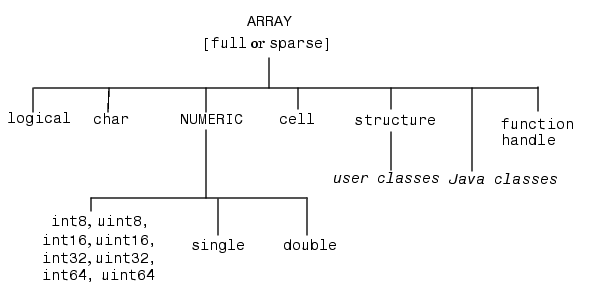ProgrammingOverview of MATLAB Data Types

There are 15 fundamental data types in MATLAB. Each of these data types is in the form of a matrix or array. This matrix or array is a minimum of 0-by-0 in size and can grow to an n-dimensional array of any size.

All of the fundamental data types are shown in lowercase text in the diagram below. Additional data types are user-defined, object-oriented `user classes` and `Java classes`. You can use the latter with the MATLAB interface to Java (see Calling Java from MATLAB in the MATLAB External Interfaces documentation).

You can create two-dimensional `double` and `logical` matrices using one of two storage formats: `full` or `sparse`. For matrices with mostly zero-valued elements, a sparse matrix requires a fraction of the storage space required for an equivalent full matrix. Sparse matrices invoke methods especially tailored to solve sparse problems.The following table describes these data types in more detail.

 Data Type Example Description `int8, uint8,int16, uint16,int32, uint32,int64, uint64` `uint16(65000)` Array of signed and unsigned integers. Requires less storage space than `single` or `double`. All integer types except for `int64` and `uint64` can be used in mathematical operations. `single` `3 * 10^38` Array of single-precision numbers. Requires less storage space than `double`, but has less precision and a smaller range. `double` `3 * 10^300``5 + 6i` Array of double-precision numbers. Two- dimensional arrays can be sparse. The default numeric type in MATLAB. `logical` `magic(4) > 10` Array of logical values of `1` or `0` to represent `true` and `false` respectively. Two-dimensional arrays can be sparse. `char` `'Hello'` Array of characters. Strings are represented as vectors of characters. For arrays containing more than one string, it is best to use cell arrays. `cell array` `a{1,1} = 12;a{1,2} = 'Red';a{1,3} = magic(4);` Array of indexed cells, each capable of storing an array of a different dimension and data type. `structure` `a.day = 12;a.color = 'Red';a.mat = magic(3);` Array of C-like structures, each structure having named fields capable of storing an array of a different dimension and data type. `function handle` `@sin` Pointer to a function. You can pass function handles to other functions. `user class` `polynom([0 -2 -5])` Objects constructed from a user-defined class. See MATLAB Classes `Java class` `java.awt.Frame` Objects constructed from a Java class. See Java Classes.Data Types Numeric Types